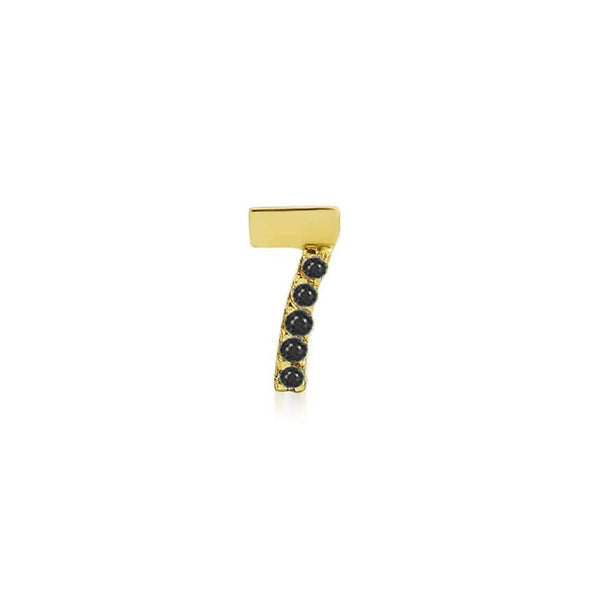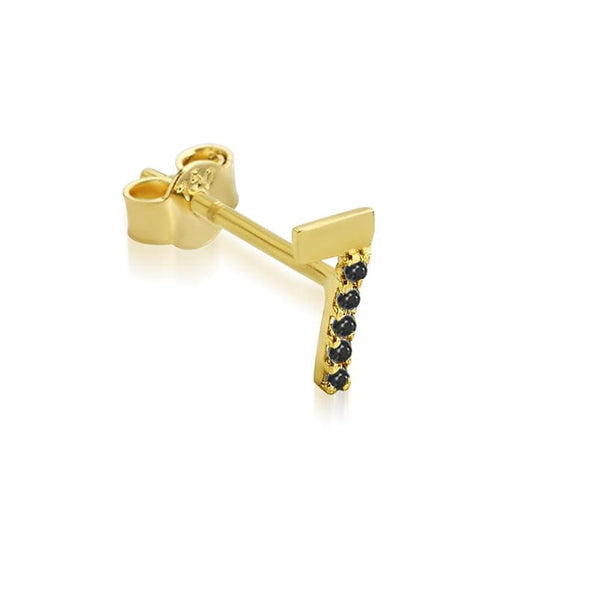# Numerology Earring 7 - Diamonds - Single

£265.00
18kt YELLOW GOLD HANDSET WITH WHITE SAPPHIRES
TOTAL LENGTH 1CM
To find your special numerology you will need to add your date of birth for the date, month and year separately till you achieve a single digit.
For example if you are born on the 18th of October 1993 your numerology calculation is as follows 1+8=9 (date), 1+0=1 (month) and 1+9+9+3=22==> 2+2=4 (year). You will need to add 9+1+4=14==> 1+4=5.